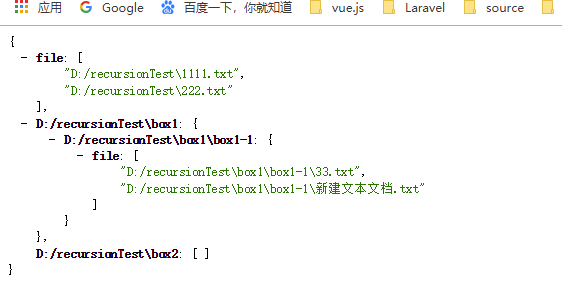• 主要介绍了c#递归遍历文件夹示例,需要的朋友可以参考下
• 易语言API无递归遍历文件夹模块源码,API无递归遍历文件夹模块,Test,FileTimeToVariantTime,EnumFile,EnumPath,newPath,newFile,EnumAllPath,FindFirstFile,FileTimeToLocalFileTime,FileTimeToSystemTime,...
• PHP递归遍历文件夹发布时间：2020-05-02 12:18:44来源：51CTO阅读：484作者：Lee_吉/*递归遍历文件夹中所有的文件*/functionget_files($dir){$files=array();if(!is_dir($dir)){return$files;}$handle=opendir($dir)...
PHP递归遍历文件夹发布时间：2020-05-02 12:18:44来源：51CTO阅读：484作者：Lee_吉/*递归遍历文件夹中所有的文件*/function get_files($dir) {$files = array();if(!is_dir($dir)) {return$files;}$handle = opendir($dir);if($handle) {while(false !== ($file = readdir($handle))) {if ($file != '.' && $file != '..') {$filename = $dir . DIRECTORY_SEPARATOR .$file;if(is_file($filename)) {if(preg_match('/.*\.php$/',$filename)){$files[] = $filename;}}else {$files = array_merge($files, get_files($filename));}}}   //  end whileclosedir($handle);}return$files;}/*递归删除文件夹中的所有文件，包括文件夹*/function emptydir($dir) {if(!is_dir($dir)) {unlink($dir);}$handle = opendir($dir);if($handle) {while(false !== ($file = readdir($handle))) {if ($file != '.' &&$file != '..') {$filename =$dir . DIRECTORY_SEPARATOR  . $file;if(is_file($filename)) {unlink($filename);}else {emptydir($filename);rmdir($filename);}}} // end whileclosedir($handle);}}
展开全文• 用python实现遍历文件夹，此函数接收一个目录，打印出目录里所有的文件（包括子目录的文件） def print_directory_contents(path): """ 这个函数接收文件夹的名称作为输入参数 返回该文件夹中文件的路径 以及其...
用python实现遍历文件夹，此函数接收一个目录，打印出目录里所有的文件（包括子目录的文件）
def print_directory_contents(path):
"""
这个函数接收文件夹的名称作为输入参数
返回该文件夹中文件的路径
以及其包含文件夹中文件的
"""
import os
for child_path in os.listdir(path):
full_child_path = os.path.join(path, child_path)
if os.path.isdir(full_child_path):
print_directory_contents(full_child_path)
else:
print(full_child_path)


转载于:https://www.cnblogs.com/zzliu/p/10662238.html
展开全文• 主要介绍了PHP递归遍历文件夹去除注释并压缩php源代码的方法,涉及php文件与目录的遍历、读取、判断及使用php_strip_whitespace函数删除注释的相关操作技巧,需要的朋友可以参考下
• 下面小编就为大家带来一篇python 递归遍历文件夹,并打印满足条件的文件路径实例。小编觉得挺不错的，现在就分享给大家，也给大家做个参考。一起跟随小编过来看看吧
•C#
• 所谓遍历(Traversal)，是指沿着某条搜索路线，依次对树中每个结点均做一次且仅做一次访问.在python中有很多方法可以快速的遍历文件夹.本文的目的是想通过遍历文件夹来充分体会递归的...知识准备2.1递归遍历文件夹...
所谓遍历(Traversal)，是指沿着某条搜索路线，依次对树中每个结点均做一次且仅做一次访问.在python中有很多方法可以快速的遍历文件夹.本文的目的是想通过遍历文件夹来充分体会递归的思想和熟练使用os模块.如有纰漏希望小伙伴们批评指正.1.需求我在桌面上有一个"test"目录,现在我要遍历它,如果遇到文件夹,就输出"xx是文件夹",遇到文件就输出"xx是文件"2.知识准备2.1递归遍历文件夹是一个简单的工作,通过for循环就可以完成,不过这里我用递归来完成它.递归就是自己调用自己,在调用一个函数的过程中，直接或间接地调用了函数本身这个就叫递归来个小例子,比如求1加到10:#求1+2+3+4+5+6+7+8+9+10#用for循环的方法完成>>> def count(num):result=0for i in range(1,num+1):result += ireturn result>>> num = count(10)>>> num55#求1+2+3+4+5+6+7+8+9+10#用递归的方法完成>>> def count(num):if num==1:return 1return count(num-1)+num>>> num = count(10)>>> num55从上面的这段代码可以看出,在函数count(),内部调用了自己也就是count函数有两点要注意的给内部调用count传入的参数是num-1,在做递归的时候就是要假设当前步骤的下一步已经实现,直接拿来用就好了.在这个例子中要实现1加到10那么我就假设1加到9已经算完了,就是count(9),那么count(9)+10就好了if num==1 这一步是给递归找出口,找不到出口就会一直调用下去,所以这一步也是很关键的.2.2 os模块的一些方法python中对文件夹的操作可以用os模块提供的一些函数,下面列出一些:os.listdir(dir_path):参数是文件夹路径,作用是把改路径下的文件夹名和文件名存入一个列表下面都是os.path下的函数os.path.exists(dir_path):参数是文件夹路径,作用是判断这个文件夹是否存在,返回布尔值os.path.join(dir_path,fileDirName):参数有二,第一个参数是文件夹路径,第二个参数是文件名.该函数的作用是拼凑文件的绝对路径os.path.isdir(abspath):判断传入的参数是否是本机上的文件夹os.path.isfile(abspath):判断传入的参数是否是文件3.实现代码#-*- coding:utf-8 -*-import os#获得一个目录下的所有的文件名和目录名(包含子目录,子文件)def getALLDirAndFile(dir_path):#判断目录是否存在,不存在直接结束函数if not os.path.exists(dir_path):print "文件不存在"return#把文件夹下的内容放入列表,便于下一步分析listName =  os.listdir(dir_path)for fileDirName in listName:#拼接成绝对路径abspath = os.path.join(dir_path,fileDirName)#print(abspath)#判断该路径是否是目录,如果是目录,就用递归的方式继续遍历,不是的话就会退出if os.path.isdir(abspath):print abspath," 是目录"#递归遍历目录getALLDirAndFile(abspath)#判断是否是文件if os.path.isfile(abspath):print abspath," 是文件"if __name__ == "__main__":getALLDirAndFile(r'C:\Users\hong\Desktop\test')结果C:\Users\hong\Desktop\test\a.html  是文件C:\Users\hong\Desktop\test\a.txt  是文件C:\Users\hong\Desktop\test\static  是目录C:\Users\hong\Desktop\test\templates  是目录C:\Users\hong\Desktop\test\templates\a.html  是文件C:\Users\hong\Desktop\test\untitled0.py  是文件
展开全文• <?.../** * Created by phpStorm. * User: BinWei * Date: 2019/6/2 ... * @description 递归遍历文件夹 * @param $dir * @return array * @author BinWei */ function recursionDir($dir...
<?php
/**
* Created by phpStorm.
* User: BinWei
* Date: 2019/6/2
* Time: 21:37
*/

/**
* @description 递归遍历文件夹
* @param $dir * @return array * @author BinWei */ function recursionDir($dir)
{
$result = [];$handle = opendir($dir); if ($handle) {
while (($file = readdir($handle)) !== false) {
if ($file != '.' &&$file != '..') {
$current_path =$dir . DIRECTORY_SEPARATOR . $file; if (is_dir($current_path)) {
$result[$current_path] = recursionDir($current_path); } else {$result['file'][] = $current_path; } } } closedir($handle);
}
return $result; }$dir = 'D:/recursionTest';

$rs = recursionDir($dir);
echo(json_encode($rs));  示例结果展开全文• 递归遍历文件夹下所有文件代码块递归遍历文件夹下所有文件package com.chow;import java.io.File; import java.util.ArrayList;/** * Created by zhouhaiming on 2017-8-3 9:20 * Email: dg_chow@163.com * * @... • EXCEL-VBA：递归遍历文件夹及子文件夹中的文件文件 • 递归遍历文件夹中的文件 思路解析 进行判断: 如果是文件夹就则转换为listFiles, 遍历数组，对每一项调用open() 如果是文件 sout public static void open(File file) { if (file.isDirectory()) { File[] ... • /*递归遍历文件夹中所有的文件*/functionget_files($dir){$files=array();if(!is_dir($dir)){return$files;}$handle=opendir($dir);if($handle){while(false!==($file=readdir($handle))){if($file!='.'&&a... • （java实习三2代码查重 相似度）递归遍历文件夹，写文本操作。 一、遍历文件夹代码模板 确定文件夹中是否存在给定文件，如果有该文件，控制台输出文件数目和路径。 import java.io.File; public class lll { static...java • boost::filesystem的使用。递归遍历文件夹执行操作。 • 遍历文件夹下面的文件夹 如果文件夹名称等于”.svn”,则修改文件夹的属性（因为”.svn”的文件都是只读的，你不能直接删除） 删除此文件夹 如果文件夹名称不等于”.svn”,则递归上面的方法 Python的实现 代码 ... • 递归遍历文件夹得到所有的目录及文件是很多面试题都会考的，所以总结一下。我的目录结构如下遍历的代码如下/*** 遍历文件夹* @param$dir* @param int $level*/function loopDir($dir){static $level = 0;$handle = ...php 生成目录树
• 递归遍历文件夹： public static void main(String[] args) {  getFile(new File("D:\\eclipsevaadinxu\\works\\test"));  }  /**  * @author admin  * @param file  * 递归遍历文件夹  */  ...java
• Java递归遍历文件夹并打印出问价内容 public static void main(String[] args) { File dir=new File("D:\\IntelliJ IDEA 2019.3.5"); printSubFile(dir); } public static void printSubFile(File dir){ File...java
• 递归遍历某个文件夹下的所有文件是一项很常见的操作，总结通过shell和Python来递归遍历文件夹的脚本如下。  shell version#!/bin/bash function walk() { for file in ls $1 do local path=$1"/"\$file ...file path filenames python shell
• 要求： 1）采用递归遍历文件夹下的所有文件，包括子文件夹下的文件 2）采用递归删除文件下的所有文件注意：以下递归删除文件的方法，只能删除
• 递归遍历文件夹，对比文件 md5 首先，需要引用 md5 的相关代码，参考这篇文章 防止链接内容被删除，这里再记录一次： md5.h #ifndef MD5_H #define MD5_H #include <string> #include <...
• 出镜率比较高的是递归遍历文件夹。其次在有的项目里，文件过大，需要进行文件分块后再进行合并。也写了一个简单的操作。遍历文件夹顺便也取出文件夹里最大文件以及最小文件。记录一下。码上 package ...java
• python递归遍历文件夹并对特定后缀文件改名 # -*- coding:utf8 -*- import os import random def rename(path): oldpath = path pos1 = path.find('_num_') pos2 = path.find('_',pos1+5) newpath = path[:pos...python...